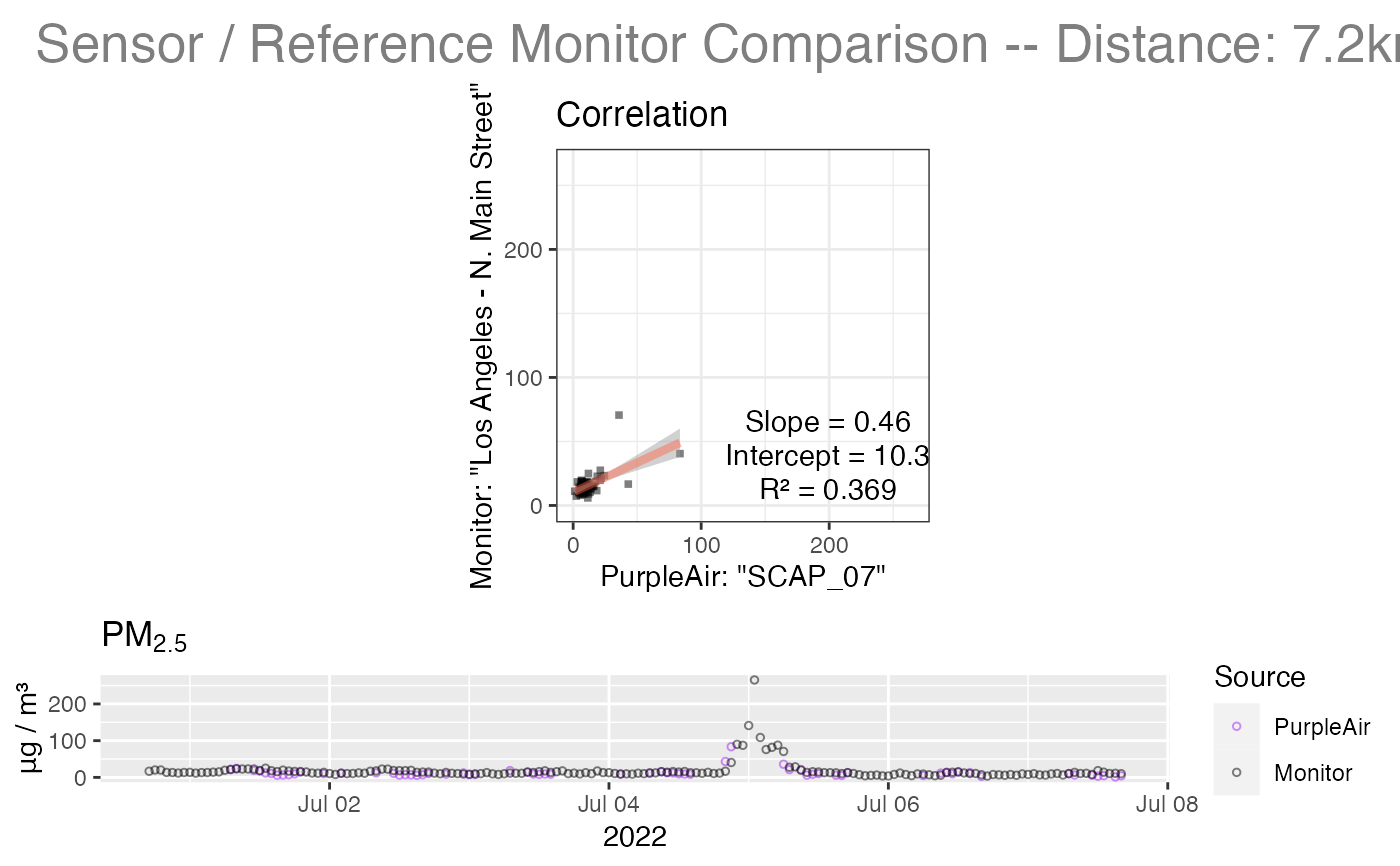Produces a linear model between data from PurpleAir and data from the closest PWFSL monitor.

A diagnostic plot is produced if showPlot = TRUE.

pat_externalFit(
pat = NULL,
showPlot = TRUE,
size = 1,
pa_color = "purple",
pwfsl_color = "black",
alpha = 0.5,
lr_shape = 15,
lr_color = "black",
lr_lwd = 1.5,
lr_lcolor = "tomato",
lr_lalpha = 0.45,
ts_shape = 1,
xylim = NULL,
channel = "ab",
replaceOutliers = TRUE,
qc_algorithm = "hourly_AB_01",
min_count = 20
)

## Arguments

pat PurpleAir Timeseries pat object. Logical specifying whether to generate a model fit plot. Size of points. Color of hourly points. Color of hourly points. Opacity of points. Symbol to use for linear model points. Color of linear model plot points. Width of linear regression line. Color of linear regression line. Opacity of linear regression line. Symbol to use for time series points. Vector of (lo,hi) limits used as limits on the correlation plot axes -- useful for zooming in. Data channel to use for PM2.5 -- one of "a", "b or "ab". Logical specifying whether or not to replace outliers. Named QC algorithm to apply to hourly aggregation stats. Aggregation bins with fewer than min_count measurements will be marked as NA.

## Value

A linear model, fitting the pat PurpleAir readings to the closest PWFSL monitor readings.

## Examples

# \donttest{
library(AirSensor)

pat_externalFit(example_pat)
#> Warning: Removed 19 rows containing non-finite values (stat_smooth).#> geom_smooth() using formula 'y ~ x'#> Warning: Removed 19 rows containing non-finite values (stat_smooth).#> Warning: Removed 19 rows containing missing values (geom_point).#> Warning: Removed 19 rows containing missing values (geom_point).# }